# How to Convet Text Date dd/mm/yy to mm/dd/yyyy as Date Format

This post explains that how to convert text date in the format dd/mm/yy to the standard date format mm/dd/yyyy in excel. How to change dates in text format dd/mm/yy to mm/dd/yyyy as date format in excel 2013.

## Convet Text dd/mm/yy to mm/dd/yyyy as Date Format

If you want to convert dates in the text format to the date format as mm/dd/yyyy, you can use a combination of the DATE function, the VALUE function, the RIGHT function, the MID function, and the LEFT function to create a new excel formula.

For example, to change dates in text format in Cell B1 to a true date format as mm/dd/yyyy (it is a standard date format in excel), you can try to run the following formula in Cell C1:

`=DATE(VALUE(RIGHT(B1,2))+2000), VALUE(MID(B1,4,2)), VALUE(LEFT(B1,2)))`

Let’s see how this formula works:

=VALUE()

The VALUE function converts a text string that represents a number to a numeric value. So this formula will convert text string returned by RIGHT, MID and LEFT functions to a numeric value.

=VALUE(RIGHT(B1,2))+2000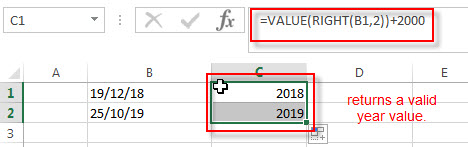The RIGHT function will extract the rightmost 2 characters from text string in Cell B1, then goes into the VALUE function to convert it as a numeric value, then add 2000 to get a valid year value. This year value will go into the DATE function as its year argument.

=VALUE(MID(B1,4,2))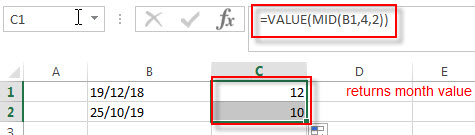The MID function will retrieve a substring from a text string at the position (start_num is 4, and num_chars is 2) in Cell B1, so it will extract two characters at the string position 4. And it will be convert to a numeric value, this value goes into the DATE function as its month argument.

=VALUE(LEFT(B1,2))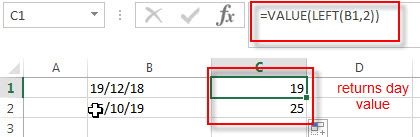The LEFT function will extract the leftmost 2 characters in Cell B1, then convert it as a numeric value by VALUE function. The returned value goes into the DATE function as its day argument.

So far, we got all three arguments (year, month, day) of DATE function from the above formulas, and then we can use the DATE function to convert it as the standard date format. Using the below formula:

=DATE(VALUE(RIGHT(B1,2)+2000), VALUE(MID(B1,4,2)), VALUE(LEFT(B1,2)))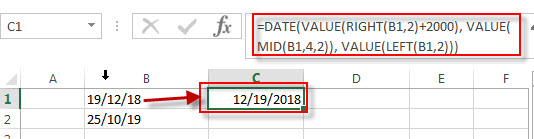You can drag fill handle over other cells which need to use this formula to convert dates in the text format to a date format mm/dd/yyyy.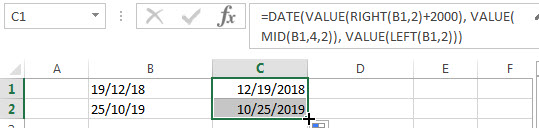Actually, you do not need to use the VALUE function in this formula because excel will convert text to numbers as necessary automatically. So we can write down the below formula:

`=DATE(RIGHT(B1,2)+2000, MID(B1,4,2), LEFT(B1,2))`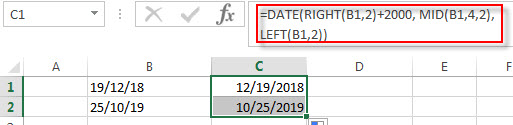### Related Formulas

• Convert date to month name with Text Function
If you want to convert the date to a month name, you can use the TEXT function with a specified format code like “mmm”in excel.  You can try to run the following TEXT formula:=TEXT(B1,”mmm”)
• Convert Date to text with Text Function in Excel
you can use TEXT function in excel to convert dates to text in a specific format code. For example, The TEXT function can use the following patterns, like as: “mm/dd/yyyy”, “yyyy/mm/dd”, etc.
• Convert date to month and year only in excel
If you want to convert the date to month and year only, you can use “yyyymm” format code within the TEXT function in excel, so you can write down the below TEXT formula:=TEXT(date,”yyyymm”)
• Convert date to month and day only in excel
If you want to convert the date (mm/dd/yyyy) to month and day only, you can use “mm dd” format code within the TEXT function in excel, so you can write down the below TEXT formula: =TEXT(date,”mm dd”)

### Related Functions

• Excel DATE function
The Excel DATE function returns the serial number for a date.The syntax of the DATE function is as below:= DATE (year, month, day)…
• Excel MID function
The Excel MID function returns a substring from a text string at the position that you specify.The syntax of the MID function is as below:= MID (text, start_num, num_chars)…
• Excel LEFT function
The Excel LEFT function returns a substring (a specified number of the characters) from a text string, starting from the leftmost character.The LEFT function is a build-in function in Microsoft Excel and it is categorized as a Text Function.The syntax of the LEFT function is as below:= LEFT(text,[num_chars])…
• Excel VALUE function
The Excel VALUE function converts a text value that represents a number to a numeric value.The syntax of the VALUE function is as below:= VALUE  (text)…
• Excel RIGHT function
The Excel RIGHT function returns a substring (a specified number of the characters) from a text string, starting from the rightmost character.The syntax of the RIGHT function is as below:= RIGHT (text,[num_chars])…